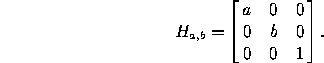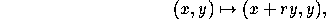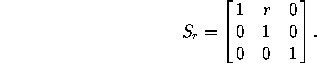2D Projective Geometry and Transformation

Jan 14, 2016

Contents

Typical Types of Transformation of 2D PlanesKey differences about projective and affine transformations:

• projective: lines mapped to lines, but parallelism may not be kept;

• affine: collinearity and parallelism are both kept

Affine Transformation

A transformation that preserves lines and parallelism (maps parallel lines to parallel lines) is an affine transformation. There are two important particular cases of such transformations:

A nonproportional scaling transformation centered at the origin has the formwhere `a,b != 0` are the scaling factors (real numbers). The corresponding matrix in homogeneous coordinates isA shear preserving horizontal lines has the formwhere `r` is the shearing factor (see Figure 1). The corresponding matrix in homogeneous coordinates isFigure 1: A shear with factor r=1/2.

Every affine transformation is obtained by composing a scaling transformation with an isometry, or a shear with a homothety and an isometry.

Projective Transformation

A transformation that maps lines to lines (but does not necessarily preserve parallelism) is a projective transformation. Any plane projective transformation can be expressed by an invertible 3×3 matrix in homogeneous coordinates:Conversely, any invertible 3×3 matrix defines a projective transformation of the plane. Projective transformations (if not affine) are not defined on all of the plane, but only on the complement of a line (the missing line is “mapped to infinity”).Figure above: In projective transformations (if not affine), a vanishing line in infinity can be warped to be a finite line.

Perspecive Transformation

The term perspecive transformation is also commonly seen. Perspective transformation projects a 3D geometric object into a 2D plane. It can be seen as a common example of projective transformation. Strictly speaking it gives a transformation from one plane to another, but if we identify the two planes by (for example) fixing a cartesian system in each, we get a projective transformation from the plane to itself, as shown in the figure below.Figure above: A perspective transformation with center O, mapping the plane P to the plane Q. The transformation is not defined on the line L, where P intersects the plane parallel to Q and going throught O.

What is the main difference between perspectivity and general projectivity?

The distinctive property of a perspectivity is thatlines joining corresponding points are concurrent. The difference between a perspectivity and projectivity is made clear by considering the composition of two perspectivities.

The composition of two perspectivities is not in general a perspectivity. However, the composition is a projectivity because a perspectivity is a projectivity, and projectivities form a group (closed), so that the composition of two projectivities is a projectivity.

This can be illustrated by the figure below:Figure above: A line projectivity. Points {a, b, c} are related to points {A, B, C} by a line-to-line perspectivity. Points {a’, b’, c’} are also related to points {A, B, C} by a perspectivity. However, points {a, b, c} are related to points {a’, b’, c’} by a projectivity; they are not related by a perspectivity because lines joining corresponding points are not concurrent. In fact the pairwise intersections result in three distinct points {p, q, r}.

1. Affine Transformations

2. Projective Transformations

3. Multiview Geometry of Computer Vision